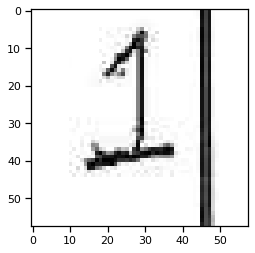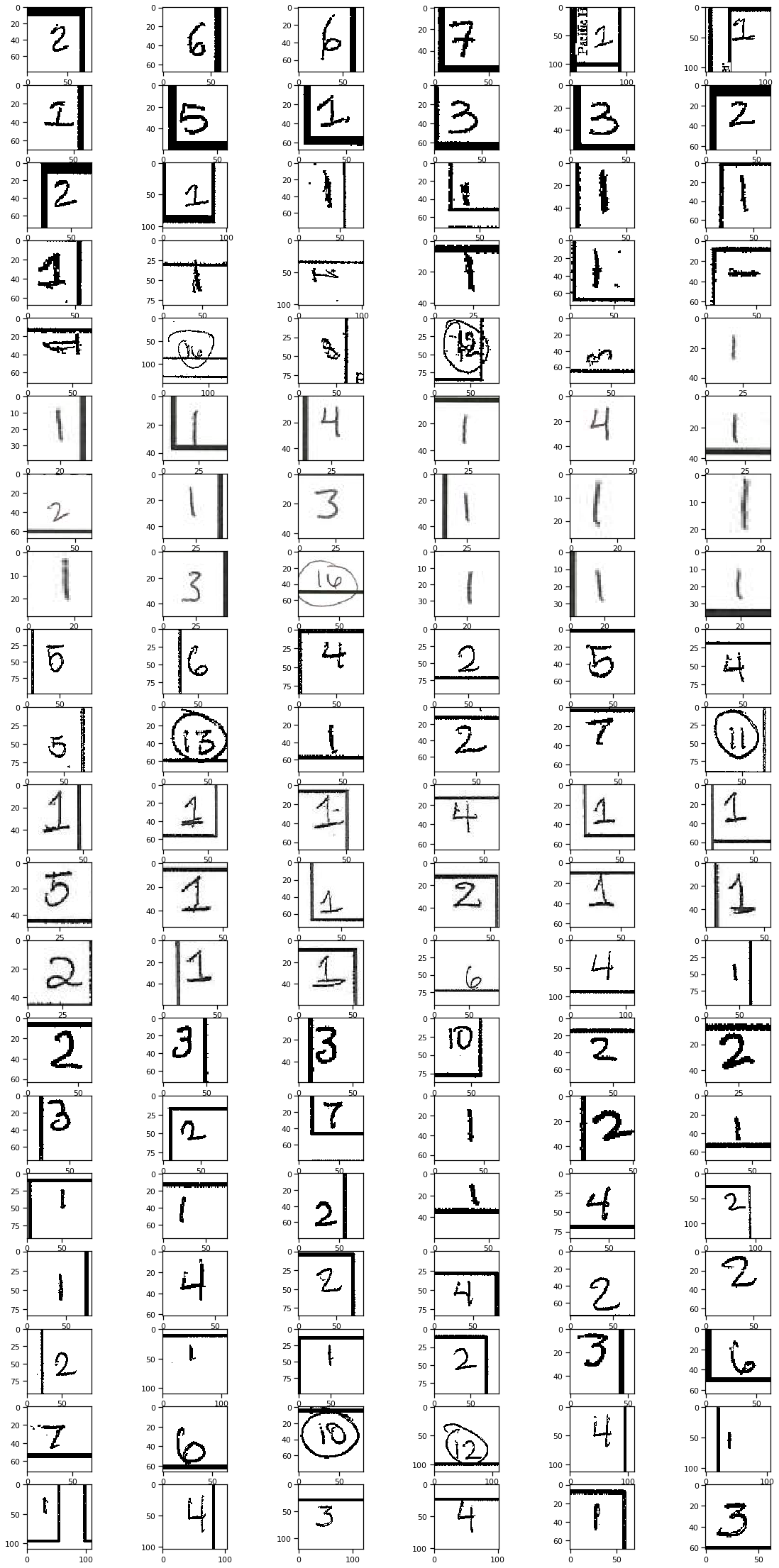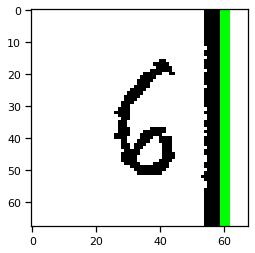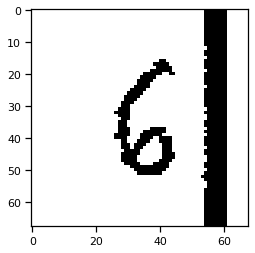# Extracting regions for handwritten marks¶

In :
import seaborn as sns
import metapack as mp
import pandas as pd
import numpy as np
import matplotlib.pyplot as plt
from IPython.display import display
from dtcv import get_image

%matplotlib inline
sns.set_context('notebook')
mp.jupyter.init()

In :
#pkg = mp.jupyter.open_package()
#pkg = mp.jupyter.open_source_package()
pkg = mp.open_package('http://library.metatab.org/sandiegodata.org-downtown_cv-5.zip')

pkg

Out:

# San Diego Downtown Homless Computer Vision Package

sandiegodata.org-downtown_cv-5 Last Update: 2019-09-13T04:52:01

Files and code for analyzing San Diego downtown homelessness data with computer vision

This dataset collects records related to a conversion of 5 years of paper maps that record positions of homeless sleepers in downtown San Diego. The San Diego Regional Data Library is converting these paper maps to a digital form with a manual process that uses an image annotation tool, and theses annotations can be used to train computer vision algorithms to georeference maps and recognize handwritten marks.

These datasets link to map urls and annotations, for three kinds of annotations:

• Ground Control Points, which identify the map image locations for known intersections, linking image coordinates ( in pixels ) to geographic coordinates.
• Image locations of handwritten marks and the number written in the mark.
• File annotations, for other handwritten notes such as the temperature and presence of rain.

• Blog Post. For more discussion about the GCP and handwritten marks, and the tasks in volved in developing computer vision algorithms for these data, see our recent blog post on the subject.
• Clustering Notebook. For some examples of using OpenCV to extract and match templates, to georeference maps, see the Templates and Clustering Jupyter Notebook].
• Extract Marks Notebook. For examples of extracting ( but not recognizing ) handwritten marks, see this notebook.

## Developer notes

After anotation JSON files are copied into S#, the list of S# urls must be updated. To refresh the list of urls run

\$  bin/update_s3.sh <s3-profile>


## Resources

In :
display(pkg.resource('counts'))
counts = pkg.resource('counts').dataframe()


### counts

http://library.metatab.org/sandiegodata.org-downtown_cv-5.zip#data%2Fcounts.csv

image_urlstringMap image URL
cxintegerX value of the center of the circle region, in pixels
cyintegerY value of the center of the circle region in pixels
rintegerRadius of the circle region, in pixels
typestringType of sleeper: Individual, Vehicle or Structure
countstringCount of sleepers
In :
# This may take a few minutes; it will download about 330 images and save them to the /tmp directory
counts['image'] = counts.image_url.apply(get_image)

In :
counts.head()
counts['count'] = pd.to_numeric(counts['count'])

In :
def crop(row):
"""Crop the handwritten mark, and hopefully the shape around it, from the image"""
x, y, r = row.cx, row.cy, row.r

r = int(r*1.0)

return row.image[y-r:y+r, x-r:x+r ]

plt.imshow(crop(counts.iloc))

Out:
<matplotlib.image.AxesImage at 0x7f91d8555550>In :
numbers = []
fig, axes = plt.subplots(nrows=20, ncols=6, figsize=(20,40))

for ax, (name, row) in zip(axes.flat, counts.iterrows()):
n = crop(row)
numbers.append(n)
ax.imshow(n)In :
import cv2

def draw_lines(img, lines):

for r,theta in lines:
a = np.cos(theta)
b = np.sin(theta)

x0 = a*r
y0 = b*r

x1 = int(x0 + 1000*(-b))
y1 = int(y0 + 1000*(a))
x2 = int(x0 - 1000*(-b))
y2 = int(y0 - 1000*(a))

cv2.line(img,(x1,y1), (x2,y2), (0,255,0),2)

img = numbers.copy()

# Apply edge detection method on the image
edges = cv2.Canny(tr,50,150,apertureSize = 3)

# This returns an array of r and theta values
lines = cv2.HoughLines(edges,1,np.pi/180, 10)

draw_lines(img, lines)

plt.imshow(img)

Out:
<matplotlib.image.AxesImage at 0x7f92a10dc390>In :
numbers = []

def invert(img):
# Convert the img to grayscale
gray = cv2.cvtColor(img,cv2.COLOR_BGR2GRAY)
_, tr = cv2.threshold (gray, 70, 255, cv2.THRESH_BINARY_INV);
return tr

for  name, row in  counts.iterrows():
numbers.append(invert(crop(row)))

In :
plt.imshow(numbers)

Out:
<matplotlib.image.AxesImage at 0x7f9207ed5978>In [ ]: